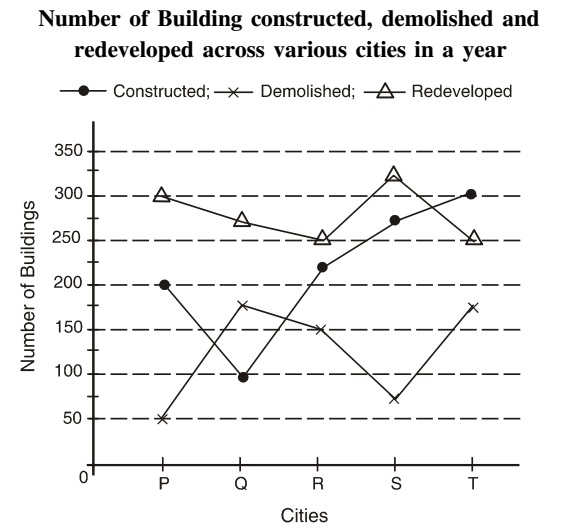## Line chart

#### Data Interpretation

Direction: Study the following graph carefully and answer the questions given below.1. What is the approximate average number of constructions (constructed, demolished and redeveloped) in the city R?

1. Average number of constructions in City R = (number of constructed building + number of demolished building + number of redeveloped building )/3

##### Correct Option: B

Average number of constructions in City R = (number of constructed building + number of demolished building + number of redeveloped building )/3
Average number of constructions in City R = 150 + 225 + 250/3 = 625/3 = 208.33 Approximate 208 %

1. What is the respective ratio of the number of building demolished in city T to the number of buildings redeveloped in city P?

1. Required ratio = number of building demolished in City T / number of buildings redeveloped in city P

##### Correct Option: D

Required ratio = number of building demolished in City T / number of buildings redeveloped in city P
Required ratio = 175 : 300 = 7 : 12

1. What is the difference between the total constructions (constructed, demolished and redeveloped) in city Q and city T?

1. Total constructions in City Q = constructed building + demolished building + redeveloped building
Total constructions in City T = constructed building + demolished building + redeveloped building

##### Correct Option: B

Total constructions in City Q = constructed building + demolished building + redeveloped building
Total constructions in City Q = 100 + 175 + 275 = 550
Total constructions in City T = constructed building + demolished building + redeveloped building
Total constructions in City T = 175 + 250 + 300 = 725
Their difference = 725 - 550 = 175

1. The total number of buildings constructed across the cities is approximately what per cent of the total number of buildings redeveloped across the cities?

1. Total number of buildings constructed across the cities = 200 + 100 + 225 + 300
Total number of buildings redeveloped across the cities = 300 + 275 + 250

##### Correct Option: C

Total number of buildings constructed across the cities = 200 + 100 + 225 + 300 = 1100
Total number of buildings redeveloped across the cities = 300 + 275 + 250 = 1400
Required percentage = 1100 x 100 /1400 = 78.57% approx. 79%

1. What is the average number of buildings demolished across the cities?

1. Average number of building = number of building across the cities (P + Q + R + S + T )/5

##### Correct Option: B

Average number of building = number of building across the cities (P + Q + R + S + T )/5
Average number of building = 50 + 175 + 150 + 75 + 175/5 = 625/5 = 125